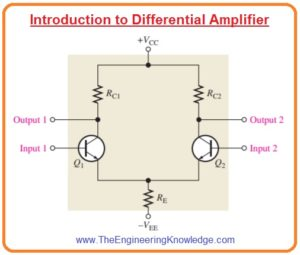Hello friends, I hope you all are doing great. In today’s tutorial, we will have a look at Introduction to Differential Amplifier. It is a type of amplifier which amplify the difference of two input signal. There are 2 modes of operation for this amplifier first is a common mode in which both inputs are the same and the second one is a differential mode in which two inputs are different.

This amplifier configuration is normally used in analogue ICs circuits arrangements. In today’s post, we will have a detailed look at its working, circuit and related parameters. So let’s get started with Introduction to Differential Amplifier.

#### Introduction to Differential Amplifier

• In below figure the basic differential amplifier circuit is shown.• You can see that this circuitry has 2 inputs and 2 outputs.
• The below figure define the dc bias analysis of differential amplifier.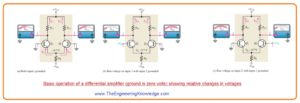• First of all the voltage across both inputs is zero volts and both emitters have voltage -0.7 volts as shown in figure denoted as (a).
• It is supposed that transistors are definatly matched with each other during construction so their dc emitter currents are at same level when thre is no voltage at inputs.

IE1=IE2

• As both emitters current linked through the reistance RE.

IE1=IE2=IRE/2

• Here

IRE=(VE-VEE)/RE

• According to estimation, we have IC=IE

IC1=IC2=IRE/2

• As both collector currents and collcetot resistances are same for zero input voltage.

VC1=VC2=VCC-IC1RC1

• This condition is defined in figure denoted as (a).
• In figure denoted as (b) input 2 is connected with the ground and positive biased voltage provided at input one.
• The positive voltage at the base of transistor Q1 increases the IC1 that decreases the VC1 and decrement in IC2 cause an increment in VC2.
• In figure denoted as (c) input, one is at connected with the ground and second input connected with the positive bias voltage.
• Positive bias voltage causes the transistor Q2 to operate more that increases current IC2.
• Also, the value of the emitter voltage is increased.
• It decreases the forward biasing of transistor Q1 as its base is connected with the ground that causes current IC1 to decrease.
• The consequence is that the increment in current IC2 generates a decrement in VC2 and the decrement in current IC1 cause voltage VC1 to increase.

#### Modes of signal operation

Single ended differential point

• When differential amplifier has such configuration in which one input is at ground level and at other input voltages is provided. It is shown in figure.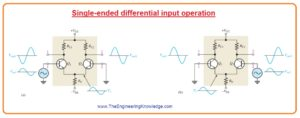• The condition when voltage is provided to first input as denoted in figure (a) inverted amplified signal voltage is shown at output one.
• With that signal voltage exits in phase at the emitter of transistor Q1.
• As the emitters of transistor Q1 and Q2 are common the signal of the emitter will be input to the transistor Q2 that operates as common emitter amplifier.
• In figure denoted as (b) signal is provided to the input two and first input is connected with the ground.
• In results, an amplified inverted signal appears at the output two.
• In this condition transistor, Q1 operates as a common base amplifier and a non-inverted signal is shown at output one.

Double-ended differential inputs

• In this input arrangements, 2 signals of opposite polarity are given to the input it shown in below figure denoted as (a).
• Every input has an effect on the output.
• Figure denoted as (b) shows the output voltage at due to signal at input one working as single-ended input.
• Figure denoted as c shows the output voltage due to the voltage at second input working as single-ended input.
• You can note that in circuits denoted as (b) and (c) polarity of a signal at input one is same.
• It also for output two both are the same polarity.
• By superimposing both outputs one voltage signals and both output 2signals you will get total output signal as shown in figure denoted as d.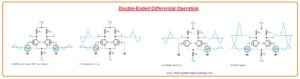Common mode input

• The most important factor of operation of differential amplifier can be seen to take into consideration common-mode condition.
• In this condition two signal voltage of similar frequency, phase and amplitude are provided to the 2 inputs of an amplifier as shown in figure denoted as (a).
•  By taking single input at one time we can understand the operation.
•  Figure denoted as (b) shows the output due to the signal only on the first input.
•  Figure denoted as (c) shows the output voltage signal due to the signal voltage at input two.
•  Note that resultant signals at on output one have opposite polarity and are on output two.
•  If input signal is given at the both of signals the output will be superimposed and resultant will be zero output voltage shown in figure denoted as d.
• This process is known as common-mode rejection.
• This mode important for such condition when an unwanted signal comes at both inputs of differential amplifiers.
• Common mode rejection mode means that the undesired signal will not be shown at the output and not disturb the original signal.
• Common mode signal or noise is the consequence of the radiated energy on the input lines from neighbouring lines such as sixty hertz or other sources.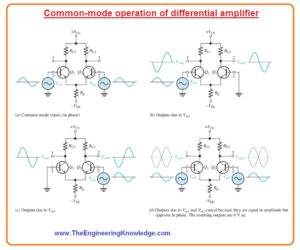#### Common mode Rejection Ratio

• Required signal is obtained at single input or one both inputs having opposite polarity.
• These required signals are get amplified and shown on the output as we have discussed above.
• Undesired signal existing at both input lines with the same polarity will cancel through the differential amplifier and not shown at the outputs.
• The measurement of amplifiers ability to reject common mode signal is a factor known as common mode rejection ratio or CMRR.
•  In ideal condition differential amplifier gives large value of gain for required signal and 0 gain for common mode signal.
• But practical differential amplifier gives very less value of common mode gain normally less than one while shows large differential voltage gain normally several thousand.
• The greater the differential gain of amplifiers with respect to common mode gain the performance of amplifier in term of rejection common mode signal.